LabVIEW 2014 Advanced Signal Processing Toolkit Help

Edition Date: June 2014

Part Number: 372656C-01

»View Product InfoDownload Help (Windows Only)

Owning Palette: Time Frequency Spectrogram VIs

Decomposes the signal into a linear combination of Gaussian chirplet elementary functions and sums the Wigner-Ville Distribution (WVD) of all the Gaussian chirplet elementary functions to compute the quadratic time-frequency representation of signal. Use this VI if the signal contains Gaussian chirps. Wire data to the signal input to determine the polymorphic instance to use or manually select the instance.

Use the pull-down menu to select an instance of this VI.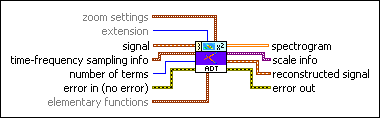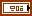zoom settings specifies the frequency zoom factor and the zoom range.zoom factor specifies how much to zoom the spectrogram. zoom factor must be an integer greater than or equal to 1. The default is 1. The larger the zoom factor, the more the detail in the spectrogram you can see.f0 specifies the lowest frequency in the resulting spectrogram in hertz. The default is NaN, which indicates that the lowest frequency equals 0 for real-valued input signals and that the lowest frequency equals –fs/2 for complex-valued input signals, where fs is the sampling rate of the signal.number of bins specifies the total number of frequency bins in the resulting spectrogram. As the value of number of bins increases, the frequency range of spectrogram increases. The default value is –1, which specifies that the frequency range of spectrogram is from f0 to fs/2, where fs is the sampling rate of the signal. number of bins must not be greater than half of zoom factor×frequency bins for real-valued input signals and must not be greater than zoom factor×frequency bins for complex-valued input signals.extension specifies the method to use to pad data at the borders of the input signal to lessen discontinuity. The extension length is half the window length.

 0 Zero padding (default)—Uses zeros to pad the input data. Watch for abrupt transitions between the padded zeros and the input data, which causes large artifacts near the transition. 1 Symmetric—Uses replications of the input data to pad the data, except that this VI left-flips the block at the input and right-flips the block at the end. 2 Periodic—Adds a replication of the input data block before and another replication after the input data block to pad the data.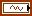signal specifies the input signal.time-frequency sampling info specifies the density to use to sample the signal in the joint time-frequency domain and defines the size of the resulting 2D time-frequency array.time steps specifies the sampling period, in samples, along the time axis in the joint time-frequency domain. The default is –1, which specifies that this VI adjusts time steps automatically so that no more than 512 rows exist in spectrogram. The number of rows in spectrogram equals the signal length divided by time steps.National Instruments recommends that you set time steps such that the number of rows in spectrogram does not exceed 512. If you specify a small value for time steps, this VI might return a large spectrogram, which requires a long computation time and more memory.If you need a small sampling period to observe more details and the signal length is large, divide the signal into smaller segments and compute the spectrogram for each segment. If the signal is oversampled, you also can downsample the signal. The scale info output contains the actual sampling period, in seconds, along the time axis.frequency bins specifies the number of bins along the frequency axis to sample the signal in the joint time-frequency domain. frequency bins must be a power of 2 and greater than 0. The scale info output contains the actual sampling period in hertz along the frequency axis.number of terms specifies the maximum number of elementary functions to use in the adaptive representation. Using more elementary functions improves the accuracy of the representation but increases computation time.error in describes error conditions that occur before this node runs. This input provides standard error in functionality.elementary functions specifies the settings of the elementary functions.type specifies the type of elementary function to use to represent the signal.

 0 Gaussian chirplet (default)—Specifies that the elementary function is the Gaussian chirplet. 1 Gaussian pulse—Specifies that the elementary function is the Gaussian pulse.max window length specifies the maximum window length of the Gaussian envelopes of the elementary functions. The default is –1, which specifies that this VI sets the maximum window length as the power of 2 that is closest to but less than the signal length. The value of max window length must be a power of 2. If the value of max window length is not a power of 2, this VI sets the value to a power of 2 automatically. Use the default setting if the size of the input signal is not greater than 1,000,000.reserved is reserved for future use.spectrogram returns the quadratic time-frequency representation of the signal. Each row corresponds to the instantaneous power spectrum at a certain time. Use the TFA Spectrogram Indicator to display the spectrogram on an intensity graph. You can save the time-dependent 2D array to a text file for use in another software environment. The resulting text file contains only Z values and does not retain the time axis information or the frequency axis information. You can use the TFA Get Time and Freq Scale Info VI to compute the time scale information and the frequency scale information of the time-frequency representation.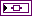scale info returns the time scale and the frequency scale information of the time-frequency representation, including the time offset, the time interval between every two contiguous rows, the frequency offset, and the frequency interval between every two contiguous columns of spectrogram. Use the TFA Get Time and Freq Scale Info VI to return detailed information about the time scale and the frequency scale.reconstructed signal returns the signal reconstructed from the detected elementary functions.error out contains error information. This output provides standard error out functionality.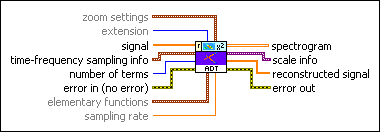zoom settings specifies the frequency zoom factor and the zoom range.zoom factor specifies how much to zoom the spectrogram. zoom factor must be an integer greater than or equal to 1. The default is 1. The larger the zoom factor, the more the detail in the spectrogram you can see.f0 specifies the lowest frequency in the resulting spectrogram in hertz. The default is NaN, which indicates that the lowest frequency equals 0 for real-valued input signals and that the lowest frequency equals –fs/2 for complex-valued input signals, where fs is the sampling rate of the signal.number of bins specifies the total number of frequency bins in the resulting spectrogram. As the value of number of bins increases, the frequency range of spectrogram increases. The default value is –1, which specifies that the frequency range of spectrogram is from f0 to fs/2, where fs is the sampling rate of the signal. number of bins must not be greater than half of zoom factor×frequency bins for real-valued input signals and must not be greater than zoom factor×frequency bins for complex-valued input signals.extension specifies the method to use to pad data at the borders of the input signal to lessen discontinuity. The extension length is half the window length.

 0 Zero padding (default)—Uses zeros to pad the input data. Watch for abrupt transitions between the padded zeros and the input data, which causes large artifacts near the transition. 1 Symmetric—Uses replications of the input data to pad the data, except that this VI left-flips the block at the input and right-flips the block at the end. 2 Periodic—Adds a replication of the input data block before and another replication after the input data block to pad the data.signal specifies the input signal.time-frequency sampling info specifies the density to use to sample the signal in the joint time-frequency domain and defines the size of the resulting 2D time-frequency array.time steps specifies the sampling period, in samples, along the time axis in the joint time-frequency domain. The default is –1, which specifies that this VI adjusts time steps automatically so that no more than 512 rows exist in spectrogram. The number of rows in spectrogram equals the signal length divided by time steps.National Instruments recommends that you set time steps such that the number of rows in spectrogram does not exceed 512. If you specify a small value for time steps, this VI might return a large spectrogram, which requires a long computation time and more memory.If you need a small sampling period to observe more details and the signal length is large, divide the signal into smaller segments and compute the spectrogram for each segment. If the signal is oversampled, you also can downsample the signal. The scale info output contains the actual sampling period, in seconds, along the time axis.frequency bins specifies the number of bins along the frequency axis to sample the signal in the joint time-frequency domain. frequency bins must be a power of 2 and greater than 0. The scale info output contains the actual sampling period in hertz along the frequency axis.number of terms specifies the maximum number of elementary functions to use in the adaptive representation. Using more elementary functions improves the accuracy of the representation but increases computation time.error in describes error conditions that occur before this node runs. This input provides standard error in functionality.elementary functions specifies the settings of the elementary functions.type specifies the type of elementary function to use to represent the signal.

 0 Gaussian chirplet (default)—Specifies that the elementary function is the Gaussian chirplet. 1 Gaussian pulse—Specifies that the elementary function is the Gaussian pulse.max window length specifies the maximum window length of the Gaussian envelopes of the elementary functions. The default is –1, which specifies that this VI sets the maximum window length as the power of 2 that is closest to but less than the signal length. The value of max window length must be a power of 2. If the value of max window length is not a power of 2, this VI sets the value to a power of 2 automatically. Use the default setting if the size of the input signal is not greater than 1,000,000.reserved is reserved for future use.sampling rate specifies the sampling rate of signal in hertz. sampling rate must be greater than 0, or this VI sets sampling rate to 1 automatically. The default is 1.spectrogram returns the quadratic time-frequency representation of the signal. Each row corresponds to the instantaneous power spectrum at a certain time. Use the TFA Spectrogram Indicator to display the spectrogram on an intensity graph. You can save the time-dependent 2D array to a text file for use in another software environment. The resulting text file contains only Z values and does not retain the time axis information or the frequency axis information. You can use the TFA Get Time and Freq Scale Info VI to compute the time scale information and the frequency scale information of the time-frequency representation.scale info returns the time scale and the frequency scale information of the time-frequency representation, including the time offset, the time interval between every two contiguous rows, the frequency offset, and the frequency interval between every two contiguous columns of spectrogram. Use the TFA Get Time and Freq Scale Info VI to return detailed information about the time scale and the frequency scale.reconstructed signal returns the signal reconstructed from the specified elementary functions.error out contains error information. This output provides standard error out functionality.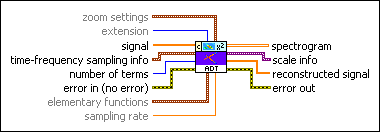zoom settings specifies the frequency zoom factor and the zoom range.zoom factor specifies how much to zoom the spectrogram. zoom factor must be an integer greater than or equal to 1. The default is 1. The larger the zoom factor, the more the detail in the spectrogram you can see.f0 specifies the lowest frequency in the resulting spectrogram in hertz. The default is NaN, which indicates that the lowest frequency equals 0 for real-valued input signals and that the lowest frequency equals –fs/2 for complex-valued input signals, where fs is the sampling rate of the signal.number of bins specifies the total number of frequency bins in the resulting spectrogram. As the value of number of bins increases, the frequency range of spectrogram increases. The default value is –1, which specifies that the frequency range of spectrogram is from f0 to fs/2, where fs is the sampling rate of the signal. number of bins must not be greater than half of zoom factor×frequency bins for real-valued input signals and must not be greater than zoom factor×frequency bins for complex-valued input signals.extension specifies the method to use to pad data at the borders of the input signal to lessen discontinuity. The extension length is half the window length.

 0 Zero padding (default)—Uses zeros to pad the input data. Watch for abrupt transitions between the padded zeros and the input data, which causes large artifacts near the transition. 1 Symmetric—Uses replications of the input data to pad the data, except that this VI left-flips the block at the input and right-flips the block at the end. 2 Periodic—Adds a replication of the input data block before and another replication after the input data block to pad the data.signal specifies the input signal.time-frequency sampling info specifies the density to use to sample the signal in the joint time-frequency domain and defines the size of the resulting 2D time-frequency array.time steps specifies the sampling period, in samples, along the time axis in the joint time-frequency domain. The default is –1, which specifies that this VI adjusts time steps automatically so that no more than 512 rows exist in spectrogram. The number of rows in spectrogram equals the signal length divided by time steps.National Instruments recommends that you set time steps such that the number of rows in spectrogram does not exceed 512. If you specify a small value for time steps, this VI might return a large spectrogram, which requires a long computation time and more memory.If you need a small sampling period to observe more details and the signal length is large, divide the signal into smaller segments and compute the spectrogram for each segment. If the signal is oversampled, you also can downsample the signal. The scale info output contains the actual sampling period, in seconds, along the time axis.frequency bins specifies the number of bins along the frequency axis to sample the signal in the joint time-frequency domain. frequency bins must be a power of 2 and greater than 0. The scale info output contains the actual sampling period in hertz along the frequency axis.number of terms specifies the maximum number of elementary functions to use in the adaptive representation. Using more elementary functions improves the accuracy of the representation but increases computation time.error in describes error conditions that occur before this node runs. This input provides standard error in functionality.elementary functions specifies the settings of the elementary functions.type specifies the type of elementary function to use to represent the signal.

 0 Gaussian chirplet (default)—Specifies that the elementary function is the Gaussian chirplet. 1 Gaussian pulse—Specifies that the elementary function is the Gaussian pulse.max window length specifies the maximum window length of the Gaussian envelopes of the elementary functions. The default is –1, which specifies that this VI sets the maximum window length as the power of 2 that is closest to but less than the signal length. The value of max window length must be a power of 2. If the value of max window length is not a power of 2, this VI sets the value to a power of 2 automatically. Use the default setting if the size of the input signal is not greater than 1,000,000.reserved is reserved for future use.sampling rate specifies the sampling rate of signal in hertz. sampling rate must be greater than 0, or this VI sets sampling rate to 1 automatically. The default is 1.spectrogram returns the quadratic time-frequency representation of the signal. Each row corresponds to the instantaneous power spectrum at a certain time. Use the TFA Spectrogram Indicator to display the spectrogram on an intensity graph. You can save the time-dependent 2D array to a text file for use in another software environment. The resulting text file contains only Z values and does not retain the time axis information or the frequency axis information. You can use the TFA Get Time and Freq Scale Info VI to compute the time scale information and the frequency scale information of the time-frequency representation.scale info returns the time scale and the frequency scale information of the time-frequency representation, including the time offset, the time interval between every two contiguous rows, the frequency offset, and the frequency interval between every two contiguous columns of spectrogram. Use the TFA Get Time and Freq Scale Info VI to return detailed information about the time scale and the frequency scale.reconstructed signal returns the signal reconstructed from the specified elementary functions.error out contains error information. This output provides standard error out functionality.